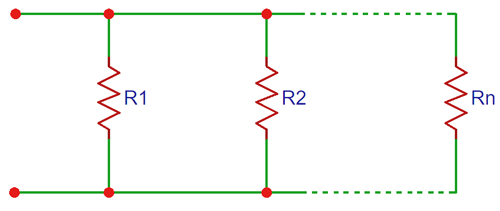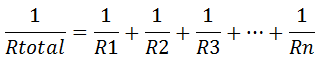# Resistors in Parallel CalculatorNo of Resistors:
 Resistor 1 OhmKilo OhmMega Ohm Resistor 2 OhmKilo OhmMega Ohm

### Working of Resistors in Parallel Calculator

This parallel resistance calculator calculates the total resistance value for all the resistors connected in parallel. Just add the number of resistors in the first column, and then enter the values of each resistor with selecting the proper unit in Ω, KΩ or MΩ.When resistors are connected in parallel, the equivalent resistor is lower than the lowest resistor among all the resistors connected in parallel. It is because when we add a new resistor in parallel, it opens a new way to pass the current so the total current after adding the new resistor is higher than the previous value, hence the total resistance is always lower than the previous one.

The formulae to calculate the total resistance in parallel, is as follows:

`1/RTotal=1/R1+1/R2+1/R3+...+1/Rn`

Here you can find the Calculator for Resistors in Series.